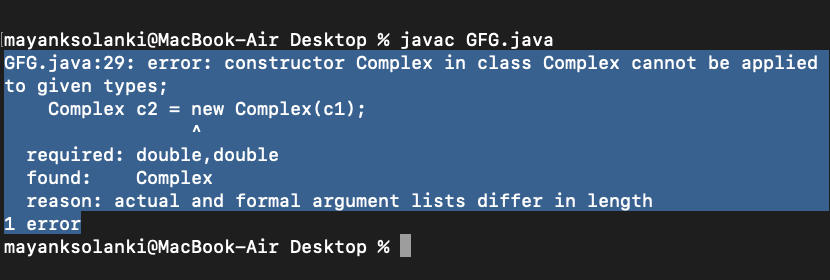Open in App
Not now

# Copy Constructor in Java

• Difficulty Level : Easy
• Last Updated : 01 Feb, 2023

Like C++, Java also supports a copy constructor. But, unlike C++, Java doesn’t create a default copy constructor if you don’t write your own. A prerequisite prior to learning copy constructors is to learn about constructors in java to deeper roots. Below is an example Java program that shows a simple use of a copy constructor.

### Here’s a basic algorithm for implementing a copy constructor in Java:

• Define a class: Create a class that represents the object you want to manage.
• Define instance variables: Within the class, define instance variables that represent the data you want to manage.
• Define a constructor: Define a constructor for the class that takes an instance of the same class as its argument. This constructor will be used to create a copy of the object.
• Initialize the instance variables: Within the constructor, initialize the instance variables with the values from the argument object.
• Use the this keyword to refer to the instance variables: To refer to the instance variables of the class within the constructor, use the this keyword.
• Check for null values: If the argument object is null, return a new instance of the class with default values for the instance variables.
• Implement deep copying: If the instance variables are objects, create new instances of those objects within the constructor and initialize them with the values from the argument object. This is called deep copying and ensures that changes to the copied object do not affect the original object.

## Java

 `class` `Person {` `    ``private` `String name;` `    ``private` `int` `age;`   `    ``public` `Person(String name, ``int` `age) {` `        ``this``.name = name;` `        ``this``.age = age;` `    ``}`   `    ``public` `Person(Person another) {` `        ``this``(another.name, another.age);` `    ``}`   `    ``// Getters and setters for the instance variables` `}`

Example 1

## Java

 `// Java Program to Illustrate Copy Constructor`   `// Class 1` `class` `Complex {`   `    ``// Class data members` `    ``private` `double` `re, im;`   `    ``// Constructor 1` `    ``// Parameterized constructor` `    ``public` `Complex(``double` `re, ``double` `im)` `    ``{`   `        ``// this keyword refers to current instance itself` `        ``this``.re = re;` `        ``this``.im = im;` `    ``}`   `    ``// Constructor 2` `    ``// Copy constructor` `    ``Complex(Complex c)` `    ``{`   `        ``System.out.println(``"Copy constructor called"``);`   `        ``re = c.re;` `        ``im = c.im;` `    ``}`   `    ``// Overriding the toString() of Object class` `    ``@Override` `public` `String toString()` `    ``{`   `        ``return` `"("` `+ re + ``" + "` `+ im + ``"i)"``;` `    ``}` `}`   `// Class 2` `// Main class` `public` `class` `Main {`   `    ``// Main driver method` `    ``public` `static` `void` `main(String[] args)` `    ``{`   `        ``// Creating object of above class` `        ``Complex c1 = ``new` `Complex(``10``, ``15``);`   `        ``// Following involves a copy constructor call` `        ``Complex c2 = ``new` `Complex(c1);`   `        ``// Note: Following doesn't involve a copy` `        ``// constructor call` `        ``// as non-primitive variables are just references.` `        ``Complex c3 = c2;`   `        ``// toString() of c2 is called here` `        ``System.out.println(c2);` `    ``}` `}`

Output

```Copy constructor called
(10.0 + 15.0i)```

Example 2

## Java

 `// Java Program to Illustrate Copy Constructor`   `// Class 1` `class` `Complex {`   `    ``// Class data members` `    ``private` `double` `re, im;`   `    ``// Constructor` `    ``public` `Complex(``double` `re, ``double` `im)` `    ``{` `        ``// this keyword refers to current instance itself` `        ``this``.re = re;` `        ``this``.im = im;` `    ``}` `}`   `// Class 2` `// Main class` `public` `class` `GFG {`   `    ``// Main driver method` `    ``public` `static` `void` `main(String[] args)` `    ``{`   `        ``// Creating object of above class` `        ``// inside main() method` `        ``Complex c1 = ``new` `Complex(``10``, ``15``);`   `        ``// Note: compiler error here` `        ``Complex c2 = ``new` `Complex(c1);` `    ``}` `}`

Output:Now, in the above code, the line calling the function with the object c1 as the parameter will give the error as the type of the parameter in the constructors is of ‘double’ type while the passed content is of ‘object’ type.